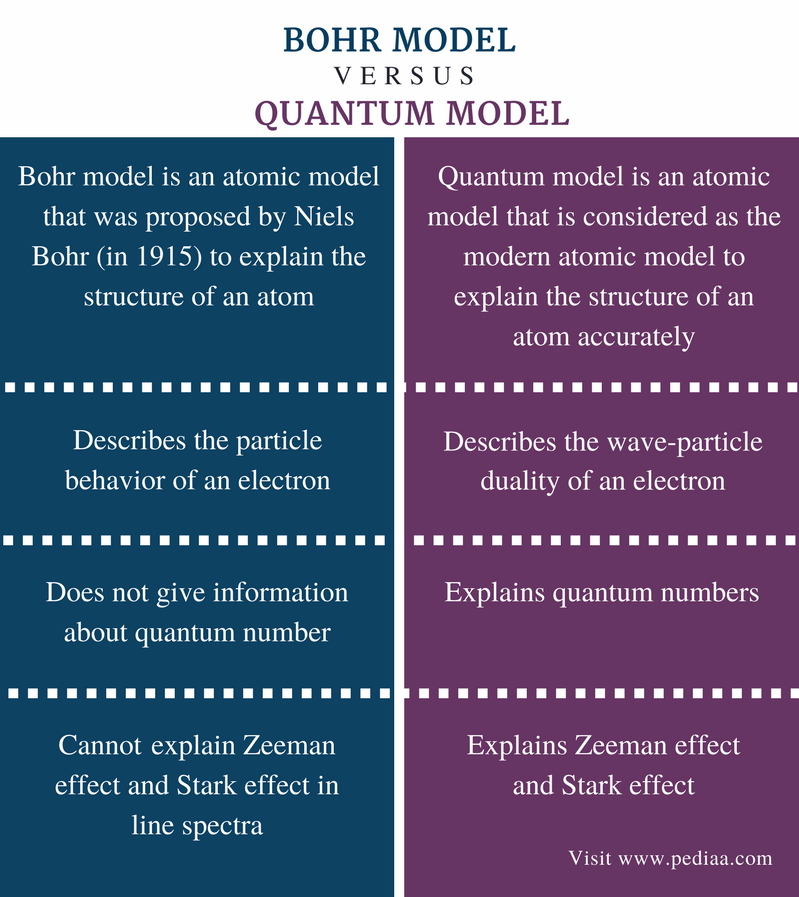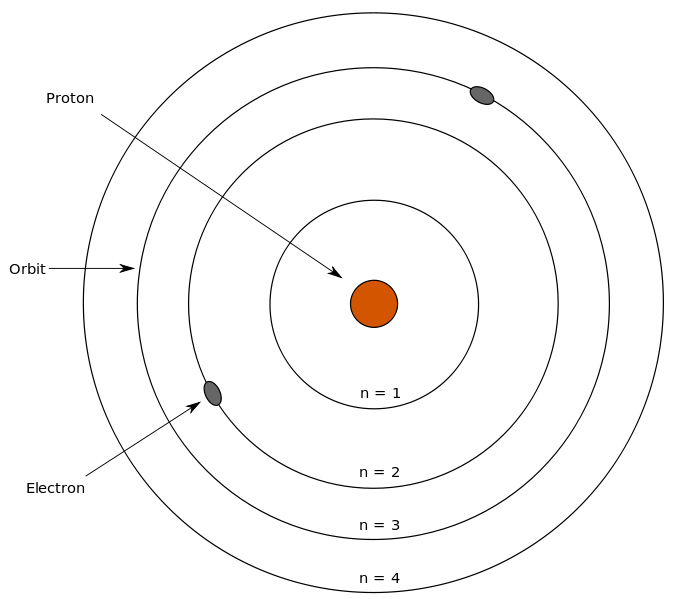# Difference Between Bohr and Quantum Model

## Main Difference – Bohr vs Quantum Model

Various scientists proposed different models to explain the structure of an atom. Bohr and quantum models are two such models. Bohr model is an advanced model, but it failed to explain some effects such as Zeeman effect and Stark effect that were observed in line spectra of large atoms. Quantum model is considered as the modern model to describe the structure of an atom. The main difference between Bohr model and Quantum model is that Bohr model explains the particle behavior of an electron whereas quantum model explains the wave-particle duality of an electron.

### Key Areas Covered

1. What is Bohr Model
– Definition, Concept, Drawbacks
2. What is Quantum Model
– Definition, Concept
3. What is the Difference Between Bohr and Quantum Model
– Comparison of Key Differences

Key Terms: Bohr Model, Electron, Electron Shells, Quantum Model, Quantum Numbers, Rutherford Model, Stark Effect, Zeeman Effect## What is Bohr Model

Bohr model is an atomic model that was proposed by Niels Bohr (in 1915) to explain the structure of an atom. It is considered as a modification of the Rutherford model. This model is more advanced than Rutherford model which does not describe the movement of electrons along electron shells around the nucleus. Bohr model also explains that these electron shells are located at discrete energy levels.

Bohr model was developed with observations of the line spectra of the hydrogen atom. Due to the presence of discrete lines in the line spectra, Bohr stated that the orbitals of an atom have fixed energies and electrons can jump from one energy level to the other while emitting or absorbing energy, resulting in a line in the line spectra.

### Concepts in Bohr Model

• The electrons move around the nucleus in spherical orbitals which have a fixed size and energy.
• The energy of an orbital is related to its size.
• The smallest orbit has the lowest energy.
• The atom is completely stable when electrons are at the lowest energy level.
• Electrons can move from one energy level to another by absorbing or releasing energy in the form of radiation.Figure 1: Bohr Model

Bohr model perfectly fits the hydrogen atom, which has a single electron and a small positively charged nucleus. But there are few drawbacks of Bohr model when explaining the atomic structure of atoms other than Hydrogen. Bohr model could not explain Zeeman effect (effect of magnetic field on the atomic spectrum) or stark effect (effect of electrical field on the atomic spectrum). This model also cannot explain the line spectra of large atoms.

## What is Quantum Model

Quantum model is an atomic model which is considered as the modern atomic model to explain the structure of an atom accurately. It can describe the effects that could not be explained by the Bohr model.

Quantum model explains the wave-particle duality of an electron. Although the quantum model is much harder to understand than Bohr model, it accurately explains observations regarding large or complex atoms. This quantum model is based on quantum theory. According to quantum theory, an electron has particle-wave duality and it is impossible to locate the exact position of the electron (uncertainty principle).Figure 2: Spatial Structure of Atomic Orbitals

It also states that orbitals are not always spherical. The orbitals have particular shapes for different energy levels and are 3D structures. According to quantum model, an electron can be given a name with the use of quantum numbers. Four types of quantum numbers are used in this:

• Principle quantum number, n (this describes the average distance of the orbital from the nucleus and the energy level.)
• Angular momentum quantum number, I (this describes the shape of the orbital.)
• Magnetic quantum number, ml (this describes the orientation of orbitals in the space.)
• Spin quantum number, ms (this describes the spinning of an electron in a magnetic field and the wave characteristics of the electron.

## Difference Between Bohr and Quantum Model

### Definition

Bohr Model: Bohr model is an atomic model that was proposed by Niels Bohr (in 1915) to explain the structure of an atom.

Quantum Model: Quantum model is an atomic model that is considered as the modern atomic model to explain the structure of an atom accurately.

### Concept

Bohr Model: Bohr model describes the particle behavior of an electron.

Quantum Model: Quantum model describes the wave-particle duality of an electron.

### Quantum Numbers

Bohr Model: Bohr model does not give information about quantum numbers.

Quantum Model: Quantum model explains quantum numbers.

### Other Effects

Bohr Model: Bohr model cannot explain Zeeman effect and Stark effect in line spectra.

Quantum Model: Quantum model explains the Zeeman effect and Stark effect.

### Conclusion

Bohr model and quantum model are two models in chemistry that are used to explain the structure of an atom. Bohr model shows some drawbacks which are explained by the quantum model. Therefore, quantum model is considered as the modern model for atomic structure. This is the difference between Bohr and quantum model.

##### References:

1. “Bohr atomic model.” Encyclopædia Britannica, inc., 5 June 2014, Available here.
2. “The Quantum Mechanical Model: Definition & Overview.” Study.com, Available here.

##### Image Courtesy:

1. “Bohr’s model” By Sharon Bewick (CC BY-SA 3.0) via Commons Wikimedia
2. “2222968” (Public Domain) via Pixabay